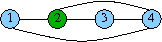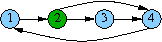TCS / Software / bliss

# The bliss graph file format

To read and save graphs in files, bliss uses a variant of the DIMACS textual graph file format. The main variation point is how the colors of graph vertices are defined, the original DIMACS format does not consider vertex colors in the same sense as bliss. A graph file in the format consists of the following four consecutive parts:
1. Comments. In the beginning of the file, there can be comment lines that start with the character "c". For instance,

`c A graph representing a Boolean circuit.`

is a comment line. Comment lines have no semantic meaning and are ignored by bliss.
2. Problem line. After the comment lines, there must be the problem definition line of form

`p edge N E`

where N is the number of vertices and E is the number of edges in the graph. In the file format, the vertices are numbered from 1 to N.
3. Vertex colors. After the problem definition line, the next lines of form

`n v c`

define the colors of the vertices, v being the number of the vertex and c its color; c should be a nonnegative integer fitting in the domain of the 'unsigned int' type in the C++ programming language. It is not necessary to include a color definition line for each vertex, the default color for a vertex is 0. If the color of a vertex is defined more than once, the last definition applies.
4. Edges. Following the color definition lines, the next E lines of form

`e v1 v2`

describe the edges in the graph, where 1≤v1,v2N are the numbers of the vertices connected by the edge. Multiple definitions of the same edge are ignored.

The graph file format itself does not make any distinction between undirected and directed graphs; this is done by a tool that uses the file format (e.g. the command `bliss graphfile` vs. `bliss -directed graphfile`). When the graph file is interpreted as an undirected graph, a line "`e v1 v2`" represents the undirected edge between the vertices v1 and v2, and is thus equivalent to the line "`e v2 v1`". For directed graphs, a line "`e v1 v2`" represents the directed edge from the vertex v1 to the vertex v2.

For instance, assuming that blue corresponds to the color "0" and green to "1", the graphcan be described in the file format as:
```c An example graph.
p edge 4 5
n 2 1
e 1 2
e 4 1
e 2 3
e 2 4
e 3 4
```
When interpreted as a directed graph, the same description represents the directed graph:[TCS main] [Contact Info] [Personnel] [Research] [Publications] [Software] [Studies] [News Archive] [Links]
Latest update: 12 March 2008. Tommi Junttila